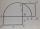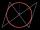# Area + The right triangle altitude theorem - math problems

#### Number of problems found: 16

• Right isosceles triangleRight isosceles triangle has an altitude x drawn from the right angle to the hypotenuse dividing it into 2 equal segments. The length of one segment is 5 cm. What is the area of the triangle?
• Right 24Right isosceles triangle has an altitude x drawn from the right angle to the hypotenuse dividing it into 2 unequal segments. The length of one segment is 5 cm. What is the area of the triangle? Thank you.
• Same areaThere is a given triangle. Construct a square of the same area.
• Conical areaA right angled triangle has sides a=12 and b=19 in right angle. The hypotenuse is c. If the triangle rotates on the c side as axis, find the volume and surface area of conical area created by this rotation.
• Hypotenuse - RTA triangle has a hypotenuse of 55 and an altitude to the hypotenuse of 33. What is the area of the triangle?
• Area of RTIn the right triangle has orthogonal projections of legs to the hypotenuse lengths 15 cm and 9 cm. Determine the area of ​​this triangle.
• Without Euclid lawsRight triangle ABC with right angle at the C has a=14 and hypotenuse c=26. Calculate the height h of this triangle without the use of Euclidean laws.
• Euklid4The legs of a right triangle have dimensions 244 m and 246 m. Calculate the length of the hypotenuse and the height of this right triangle.
• Sides of the triangleCalculate triangle sides where its area is S = 84 cm2 and a = x, b = x + 1, xc = x + 2
• Area of RTCalculate the right triangle area that hypotenuse has length 14, and one hypotenuse segment has length 5.
• Right ΔA right triangle has the length of one leg 11 cm and the hypotenuse 61 cm size. Calculate the height of the triangle.
• Isosceles triangle 9Given an isosceles triangle ABC where AB= AC. The perimeter is 64cm, and the altitude is 24cm. Find the area of the isosceles triangle.
• Circle in rhombusIn the rhombus is an inscribed circle. Contact points of touch divide the sides to parts of length 19 cm and 6 cm. Calculate the circle area.
• Free space in the gardenThe grandfather's free space in the garden was in the shape of a rectangular triangle with 5 meters and 12 meters in length. He decided to divide it into two parts and the height of the hypotenuse. For the smaller part creates a rock garden, for the large
• Squares above sidesTwo squares are constructed on two sides of the ABC triangle. The square area above the BC side is 25 cm2. The height vc to the side AB is 3 cm long. The heel P of height vc divides the AB side in a 2: 1 ratio. The AC side is longer than the BC side. Calc
• Goat and circlesWhat is the radius of a circle centered on the other circle and the intersection of the two circles is equal to half the area of the first circle? This task is the mathematical expression of the role of agriculture. The farmer has circular land on which g

Do you have an interesting mathematical word problem that you can't solve it? Submit a math problem, and we can try to solve it.

We will send a solution to your e-mail address. Solved examples are also published here. Please enter the e-mail correctly and check whether you don't have a full mailbox.

Please do not submit problems from current active competitions such as Mathematical Olympiad, correspondence seminars etc...

Area - math problems. The right triangle altitude theorem - math problems.